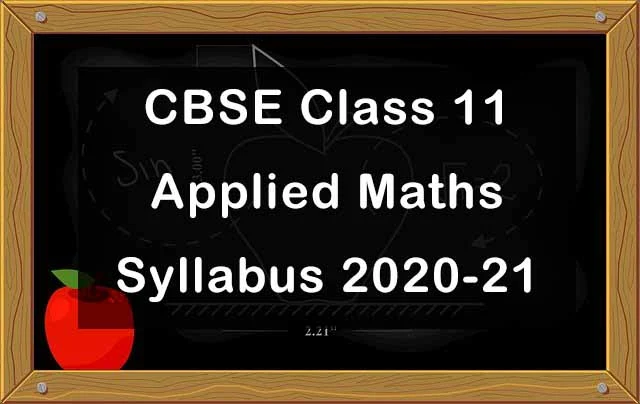## CBSE Class 11 Applied Maths Syllabus 2020-21| Check Latest Exam Pattern

CBSE has released Class 11 Applied Maths Syllabus 2020-21 which is important for knowing essential topics for examination purpose. Due to ongoing health crisis, in this academic session the board has decided to revise the syllabus with the objective to ease the pressure on the students. The question paper will be of 80 marks while internal assessment will be of 20 marks.Units Marks Numbers, Quantification and Numerical Applications 09 Algebra 10 Mathematical Reasoning 06 Calculus 10 Probability 10 Descriptive Statistics 12 Basics of Financial Mathematics 18 Coordinate Geometry 05 Total 80 Internal Assessment 20

Unit I Numbers, Quantification and Numerical Applications

• Binary Numbers
• Indices, Logarithm and Antilogarithm
• Laws and properties of logarithms
• Simple applications of logarithm and antilogarithm
• Numerical problems on averages, calendar, clock, time, work and distance, mensuration, seating arrangement

Unit II Algebra

• Sets
• Types of sets
• Venn diagram
• De Morgan's laws
• Problem solving using Venn diagram
• Relations and types of relations
• Introduction of Sequences, Series
• Arithmetic and Geometric progression
• Relationship between AM and GM
• Basic concepts of Permutations and Combinations
• Permutations
• Combinations with standard results

Unit III Mathematical and Logical Reasoning

• Mathematically acceptable statements
• Connecting words/ phrases in Mathematical statement consolidating the understanding of "if and only if (necessary and sufficient) condition", "implies", "and/or", "implied by", "and", "or", "there exists" and their use through variety of examples related to real life and Mathematics.
• Problems based on logical reasoning (coding-decoding, odd man out, blood relation, syllogism etc)

Unit IV Calculus

• Introducing functions
• Domain and Range of a function
• Types of functions (Polynomial function; Rational function; Logarithm function; Exponential function; Modulus function; Greatest Integer function, Signum function)
• Graphical representation of functions
• Concept of limits and continuity of a function
• Instantaneous rates of change
• Differentiation as a process of finding derivative
• Derivatives of algebraic functions using Chain rule

Unit V Probability

• Random experiment, sample space, events, mutually exclusive events
• Independent and Dependent Events
• Law of Total Probability
• Bayes’ Theorem

Unit VI Descriptive Statistics

• Data on various scales (nominal, ordinal, interval and ratio scale)
• Data representation and visualization
• Data interpretation ( dispersion, deviation, variance, skewness and kurtosis)
• Percentile rank and quartile rank
• Correlation (Pearson and Spearman method of correlation)
• Applications of descriptive statistics using real time data

Unit VII Basics of Financial Mathematics

• Interest and interest rate
• Accumulation with simple and compound interest
• Simple and compound interest rates with equivalency
• Effective rate of interest
• Present value, net present value and future value
• Annuities, calculating value of regular annuity
• Simple applications of regular annuities
• Tax, calculation of tax and simple applications of tax calculation in Goods and service tax, Income Tax
• Bills, tariff rates, fixed charge, surcharge, service charge
• Calculation and interpretation of electricity bill, water supply bill and other supply bills
(Comparing interest rates on various types of savings; calculating income tax; electricity bills, water bill; service surcharge using realistic data)

Unit VIII Coordinate Geometry

• Straight Line
• Circles

Deleted Topics from Class 11 Applied Maths Syllabus 2020-21

Unit I: Numbers, Quantification and Numerical Applications

• Prime Numbers, Encryptions using Prime Numbers
• Complex Numbers (Preliminary idea only)

Unit II: Algebra

• Circular Permutations, Permutations with restrictions
• Combinations when repetition is allowed

Unit III: Mathematical and Logical Reasoning

• No deletion

Unit IV: Calculus

• Composite functions
• Tangent line and equations of tangents

Unit V: Probability

• No deletion

Unit VI: Descriptive Statistics

• Types of data (raw data, univariate data, bivariate and multi-variate data)
• Data interpretation (central tendency only)

Unit VII Basics of Financial Mathematics

• No deletion

Unit VIII Coordinate Geometry

• Parabola
(only standard forms and graphical representation on two-dimensional plane)